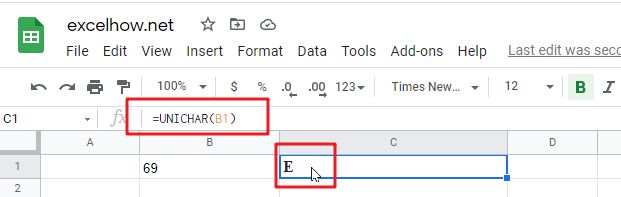# ExcelHow

This post will guide you how to use Google Sheets UNICHAR function with syntax and examples.

## Description

The Google Sheets UNICHAR function returns the Unicode character for a given number. This function support to return characters in both the UTF-8 and UTF-16 character set.

The UNICHAR function can be used to returns a Unicode character based on a given number in google sheets.  The purpose of this function is to get Unicode character by a number and its returned value is a Unicode character.

The UNICHAR function is a build-in function in Google Sheets and it is categorized as a Text Function.

## Syntax

The syntax of the UNICHAR function is as below:

= UNICHAR(number)

Where the UNICHAR function argument is:

• number -This is a required argument. The number to convert into a Unicode character.

Note:

• Unicode is a character set. It is a very large character set. And it contains 137439 characters.
• If number argument is 0, the UNICHAR function returns a #VALUE! Error message.
• If the number does not have a corresponding Unicode character, and the UNICHAR function returns a #VALUE! Error.

## Google Sheets UNICHAR Function Examples

The below examples will show you how to use google sheets UNICHAR function to convert a number to Unicode character.

#1 To get a Unicode character for a number value in cell B1, just using the following formula:

`=UNICHAR(B1)  //it returns E`